# Pipes

Water pipe has a cross-section 1087 cm2. An hour has passed 960 m3 of water. How much water flows through the pipe with cross-section 300 cm2 per 9 hours if water flow same speed?

Correct result:

V2 =  2384.5 m3

#### Solution: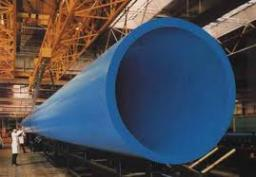We would be pleased if you find an error in the word problem, spelling mistakes, or inaccuracies and send it to us. Thank you!Tips to related online calculators
Check out our ratio calculator.
Tip: Our volume units converter will help you with the conversion of volume units.
Do you want to convert velocity (speed) units?

## Next similar math problems:

• ExaminationThe class is 21 students. How many ways can choose two to examination?
• Gasoline canisters35 liters of gasoline is to be divided into 4 canisters so that in the third canister will have 5 liters less than in the first canister, the fourth canister 10 liters more than the third canister and the second canister half of that in the first canister
• Percentage 1052 shorts and missed 13. Calculate percentage
• Today in schoolThere are 9 girls and 11 boys in the class today. What is the probability that Suzan will go to the board today?
• BottlesThe must is sold in 5-liter and 2-liter bottles. Mr Kucera bought a total of 216 liters in 60 bottles. How many liters did Mr. Kucera buy in five-liter bottles?
• Hectoliters of waterThe pool has a total of 126 hectoliters of water. The first pump draws 2.1 liters of water per second. A second pump pumps 3.5 liters of water per second. How long will it take both pumps to drain four-fifths of the water at the same time?
• Train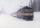The train passes part of the line for 95 minutes at speed 75 km/h. What speed would have to go in order to shorten the driving time of 20 minutes?
• Tractors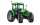Wheel and belt tractors each day plow 8 ha of fields. For plow 76 hectares, the wheel would have to work for 12 days and 8 days with the belt tractor together. How many hectares do each day of each tractor?
• Cows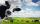4 cows spent 16 bags of hay in 5 days. How many bags of hay sacks is needed for 5 cows for seven days?
• Speedometer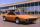The speedometer of a car reads 66 km/h. It's accurate to within 4.1% What is the maximum possible error?
• Barrel with water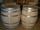The water barrel has a weight of 52 kg. When we pour a quarter of the water, the weight of the barrel is reduced to 40 kg. Determine the weight of the empty barrel.
• Gas consumption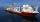The vessel consume 100 tons of gas in 250 miles. How many fuel will the vessel consume if it travels 400 miles?
• Velocity ratio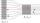Determine the ratio at which the fluid velocity in different parts of the pipeline (one part has a diameter of 5 cm and the other has a diameter of 3 cm), when you know that at every point of the liquid is the product of the area of tube [S] and the fluid
• Tmp workers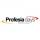Three temporary workers cleaned 60 m of walkway in 5 hours. How many meters of the walkway cleared seven volunteers for eight hours?
• Numbers at ratio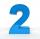The two numbers are in a ratio 3:2. If we each increase by 5 would be at a ratio of 4:3. What is the sum of original numbers?
• Liters od milkThe cylinder-shaped container contains 80 liters of milk. Milk level is 45 cm. How much milk will in the container, if level raise to height 72 cm?
• Cylinder melted into cuboid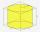A circular cylinder has area of cross section 56cm2 and the height is 10cm the cylinder is melted and made into a cuboid of base area 16cm2. What is the height of the cuboid?